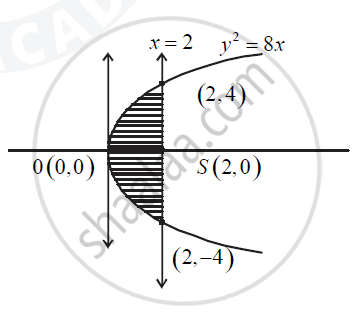ISC (Commerce) Class 12CISCE
Share

# Draw a Rough Sketch of the Curve and Find the Area of the Region Bounded by Curve Y2 = 8x and the Line X =2. - ISC (Commerce) Class 12 - Mathematics

ConceptArea of the Region Bounded by a Curve and a Line

#### Question

Draw a rough sketch of the curve and find the area of the region bounded by curve y2 = 8x and the line x =2.

#### SolutionGiven equation is y2 = 8x

Comparing with y2 -4ax

we get 4a = 8

i.e a  = 2

Given y^2 = 4(2)x

y^2 = 8x

:. y = sqrt(8x)

Also x = 2 meets y^2 = 8x

:. y^2 = 16

:. y = +-4

:. (2,4) and (2,-4) are their point of intersection

:. Required are A = 2int_0^2 sqrt(8x) dx

=2sqrt8 int_0^2 x^("1/2") dx

= 4sqrt2 = [x^("3/2")/("3/2")]_0^2

= (8sqrt2)/3 [2^"3/2" - 0]

= (8sqrt2)/3 xx sqrt8

= (8sqrt2xx2sqrt2)/3 = 32/3` sq.units

Is there an error in this question or solution?

#### Video TutorialsVIEW ALL 

Solution Draw a Rough Sketch of the Curve and Find the Area of the Region Bounded by Curve Y2 = 8x and the Line X =2. Concept: Area of the Region Bounded by a Curve and a Line.
S